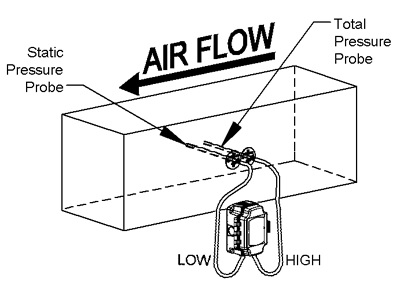# How to Determine Flow Velocity by Pitot Tube Air Flow Velocity Transmitter?

Air Flow Velocity by Pitot tube was invented by the French physicist h. Pinto in the 18th century. A simple pitot tube has a metal tube with a small hole at the end as a pressure guide tube, which is measuring the total pressure of the fluid in the direction opposite to the flow beam; another pressure guide tube is led out on the main pipe wall near the front of the metal tube to measure the static pressure.

The differential pressure gauge is connected with two pressure pipes, and the measured pressure is the dynamic pressure. According to Bernoulli's theorem, the dynamic pressure is directly proportional to the square of the velocity. Therefore, the velocity of fluid can be measured by pitot tube. After improving the structure, it becomes a combined pitot tube, namely Pitot static pressure tube. It is a double-layer pipe bent at right angles. The outer sleeve is sealed with the inner sleeve, and there are several small holes around the outer sleeve. When measuring, insert the sleeve into the middle of the pipe under test. The orifice of the inner casing is facing the direction of the flow beam, and the orifice of the small hole around the outer casing is just perpendicular to the direction of the flow beam.Principle of Pitoty Type Air Flow Velocity Transmitter

A Total Pressure Probe, aligned into the airflow, senses the duct velocity pressure and the static pressure, which equals the total pressure. A Static Pressure Probe aligned at a right angle to the airflow senses only the static pressure. The difference between the total pressure reading and the static pressure reading is the Velocity Pressure.

If you connect the Total Pressure Probe to the HIGH port on a differential pressure sensor and the Static Pressure Probe to the LOW port on the differential pressure sensor, then the sensor's output will be the Velocity Pressure.At this time, the flow velocity of the fluid at this point can be calculated by measuring the pressure difference between the inner casing and the outer casing.

GE-374 Air Flow Velocity Transmitter is designed for air velocity measuring, CE certificate, ATEX Explosion Proof is available, monitoring and controll...

The Flow Velocity is then determined with the following equation:
V = 4005 x √ΔP
V = Flow Velocity in feet per minute.
√= Square root of the number to the right .
ΔP = The Velocity Pressure measured by the pressure sensor

Example: Measuring a Velocity Pressure of .75”W.C. equals a Flow Velocity of 3,468 Ft/Min.

V = 4005 x √0.75
√0.75 = 0.866   •   4005 x 0.866 = 3,468   •   Flow Velocity = 3,468 Ft/Min

Determining air flow is a matter of multiplying the cross sectional area of a duct by the air velocity. If the dimensions of the duct are known, then the cross-sectional area can be easily determined and the volumetric flow calculated. The one thing to keep in mind is that the air velocity is not uniform at all points of the duct. This is true because the velocity is lowest at the sides where the air is slowed down by friction. To account for this, using an averaging Pitot tube with multiple sensing points will more accurately reflect the average velocity.

ATEX Explosion Proof Air Flow Velocity Transmitter, Pitot type, max temperature 400C, Measure the flow ...

Q (air flow rate)= A (area of duct) * V (air velocity)